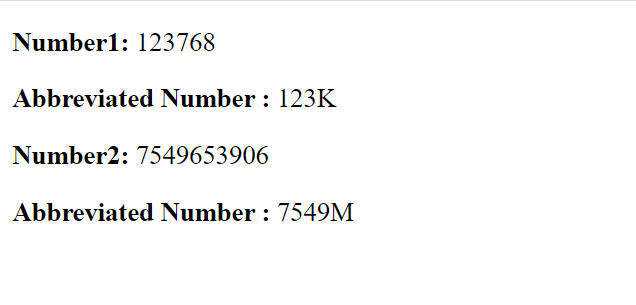Related Articles

# Abbreviate number in Kilo(K) and Million(M) using filter in Vue.js

• Last Updated : 17 Mar, 2021

In this article, we are going to learn how to abbreviate a number using filters in VueJS. Filters are a functionality provided by Vue components that let you apply formatting and transformations to any part of your template dynamic data.

Filters are a functionality provided by Vue components that let you apply formatting and transformations to any part of your template dynamic data. The filter property of the component is an object. A single filter is a function that accepts a value and returns another value. The returned value is the one that’s actually printed in the Vue.js template.

The abbreviation of numbers in kilo(K) and million(M) can be performed using filters. We first check if all the number is less than one million or greater. This can be done by using simple math logic or counting the length of the number by converting it to a string. If the number is less than a million then we divide it by 1000, otherwise, we divide the number by 1000000 and return the floor value of it along with the abbreviation needed.

Example:

## index.html

 `<``html``>``<``head``>``    ``<``script` `src``=``"https://cdn.jsdelivr.net/npm/vue@2/dist/vue.js"``>``    ``````<``body``>``    ``<``div` `id``=``'parent'``>``        ``<``p``><``strong``>Number1: ``          ``{{number1}}``        ````        ``<``p``><``strong``>``          ``Abbreviated Number : ``          ``{{ number1 | abbr }}``        ````        ``<``p``><``strong``>``          ``Number2: {{number2}}``        ````        ``<``p``><``strong``>``          ``Abbreviated Number : ``          ``{{ number2 | abbr }}``        ````    ````    ``<``script` `src``=``'app.js'``>`````

## app.js

 `const parent = ``new` `Vue({``    ``el: ``'#parent'``,``    ``data: {``        ``number1: 123768,``        ``number2: 7549653906``    ``},`` ` `    ``filters: {``        ``abbr: ``function``(num) {``            ``if` `(String(num).length < 7) {``                ``return` `Math.floor(num/1000) + ``'K'``;``            ``} ``else` `{``                ``return` `Math.floor(num/1000000) + ``'M'``;``            ``}``        ``}``    ``}``})`

Output:My Personal Notes arrow_drop_up